# Circuits II EE 221 Unit 6 Instructor Kevin

• Slides: 9Circuits II EE 221 Unit 6 Instructor: Kevin D. Donohue Active Filters, Connections of Filters, and Midterm ProjectLoad Effects Ø Ø If the output of the filter is not buffered, then for different loads, the frequency characteristics of the filter will change. Example: Both low-pass filters below have fc = 1 k. Hz and GDC = 1 (or 0 d. B). Find: fc and GDC when a 100 load is placed across the output vo(t). Result: fc = 11 k. Hz and GDC = 1/11 (or -21 d. B) for the passive circuit, while fc and GDC remain unchanged for the active circuit.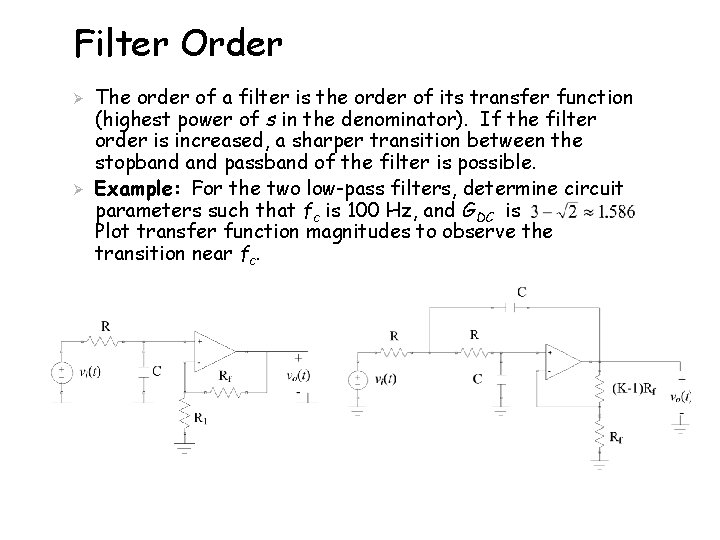Filter Order Ø Ø The order of a filter is the order of its transfer function (highest power of s in the denominator). If the filter order is increased, a sharper transition between the stopband passband of the filter is possible. Example: For the two low-pass filters, determine circuit parameters such that fc is 100 Hz, and GDC is Plot transfer function magnitudes to observe the transition near fc.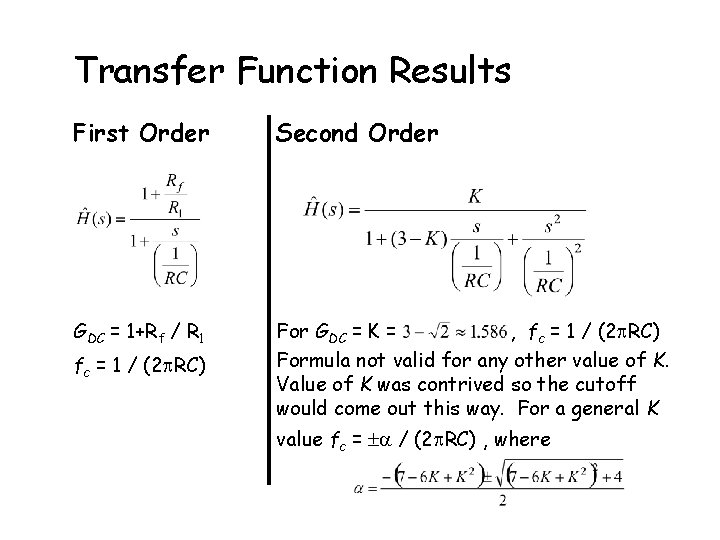Transfer Function Results First Order Second Order GDC = 1+Rf / R 1 For GDC = K = , fc = 1 / (2 RC) Formula not valid for any other value of K. Value of K was contrived so the cutoff would come out this way. For a general K fc = 1 / (2 RC) value fc = / (2 RC) , where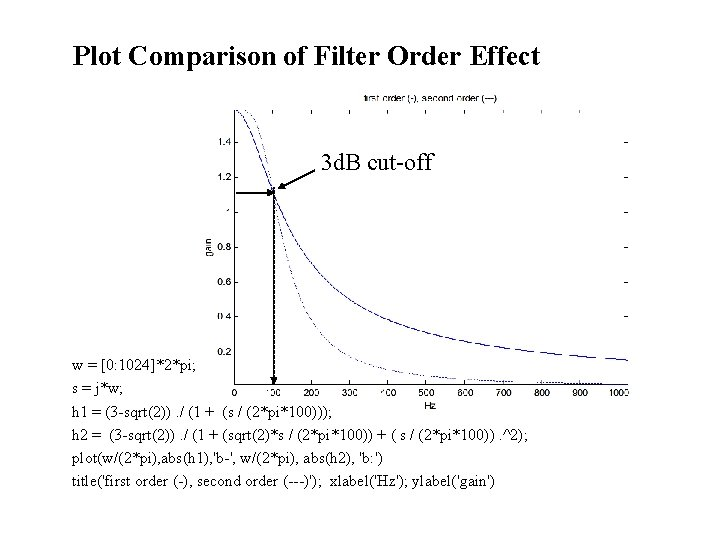Plot Comparison of Filter Order Effect 3 d. B cut-off w = [0: 1024]*2*pi; s = j*w; h 1 = (3 -sqrt(2)). / (1 + (s / (2*pi*100))); h 2 = (3 -sqrt(2)). / (1 + (sqrt(2)*s / (2*pi*100)) + ( s / (2*pi*100)). ^2); plot(w/(2*pi), abs(h 1), 'b-', w/(2*pi), abs(h 2), 'b: ') title('first order (-), second order (---)'); xlabel('Hz'); ylabel('gain')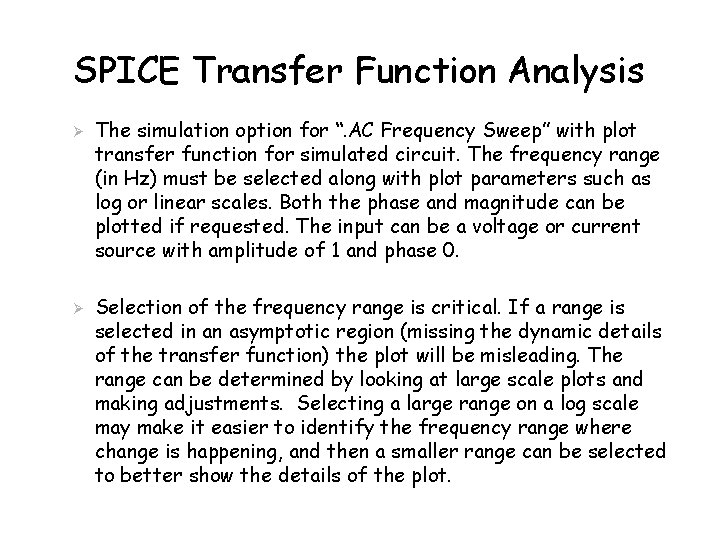SPICE Transfer Function Analysis Ø Ø The simulation option for “. AC Frequency Sweep” with plot transfer function for simulated circuit. The frequency range (in Hz) must be selected along with plot parameters such as log or linear scales. Both the phase and magnitude can be plotted if requested. The input can be a voltage or current source with amplitude of 1 and phase 0. Selection of the frequency range is critical. If a range is selected in an asymptotic region (missing the dynamic details of the transfer function) the plot will be misleading. The range can be determined by looking at large scale plots and making adjustments. Selecting a large range on a log scale may make it easier to identify the frequency range where change is happening, and then a smaller range can be selected to better show the details of the plot.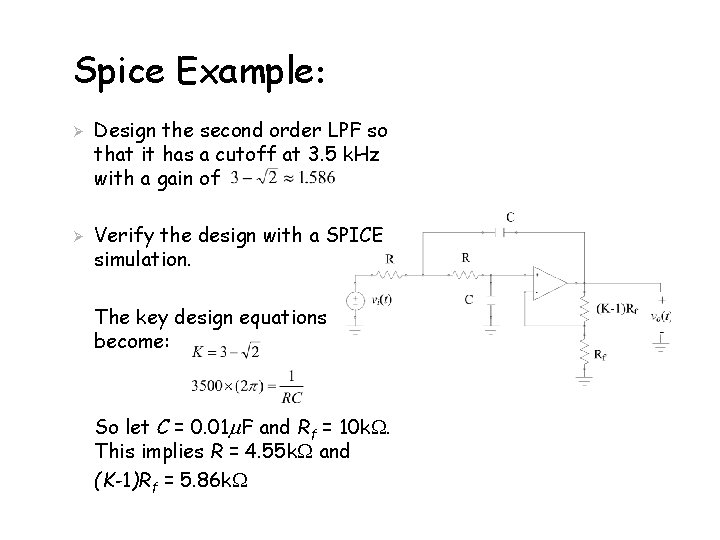Spice Example: Ø Ø Design the second order LPF so that it has a cutoff at 3. 5 k. Hz with a gain of Verify the design with a SPICE simulation. The key design equations become: So let C = 0. 01 F and Rf = 10 k. This implies R = 4. 55 k and (K-1)Rf = 5. 86 kSPICE Results (Plot range 10 to 10 MHz) Ø The magnitude plot in d. B. Crosshair marker near 3 d. B cutoff (What is happening near 100 k. Hz)?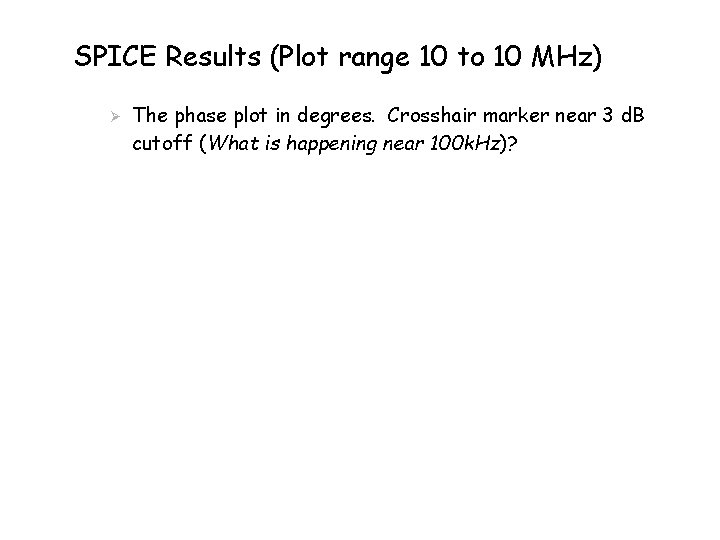SPICE Results (Plot range 10 to 10 MHz) Ø The phase plot in degrees. Crosshair marker near 3 d. B cutoff (What is happening near 100 k. Hz)?